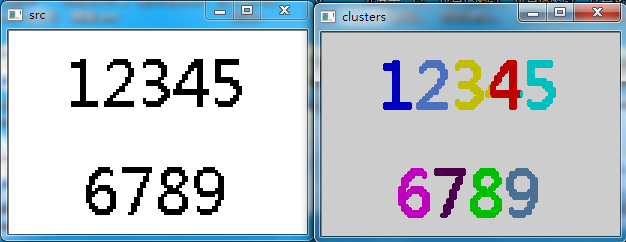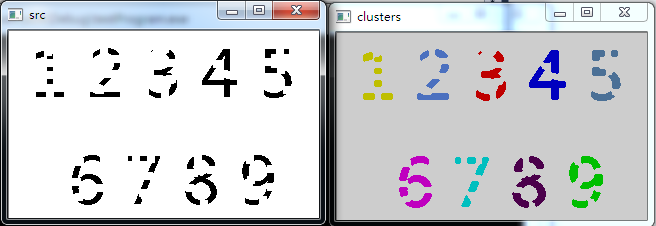• 机器翻译中要计算未对齐句对的翻译概率，我们可以采用EM算法获取   P(f|e) =Sigma(P(a, f|e))，一共有如下3种对齐方式       初始化设定 t(x|b)=t(x|c)=t(y|b)=t(y|c)=1/2   对齐1：p(a,f|e)=1/2*1/2=1...
一

机器翻译中要计算未对齐句对的翻译概率，我们可以采用EM算法获取

P(f|e) =Sigma(P(a, f|e))，一共有如下3种对齐方式初始化设定 t(x|b)=t(x|c)=t(y|b)=t(y|c)=1/2

对齐1：p(a,f|e)=1/2*1/2=1/4
对齐2：p(a,f|e)=1/2*1/2=1/4
对齐3：p(a,f|e)=1/2

继续计算

对齐1：p(a|e,f)=(1/4)/(1/4+1/4)=1/2
对齐2：p(a|e,f)=(1/4)/(1/4+1/4)=1/2
对齐3：p(a|e,f)=(1/2)/(1/2)=1

tc(x|b)=1/2
tc(x|c)=1/2
tc(y|b)=1+1/2=3/2
tc(y|c)=1/2

完成E步骤，利用E步骤获取的信息重新估计参数

t(x|b)=(1/2)/(1/2+3/2)=1/4
t(x|c)=(1/2)/(1/2+1/2)=1/2
t(y|b)=(3/2)/(1/2+3/2)=3/4
t(y|c)=(1/2)/(1/2+1/2)=1/2

完成M步骤，重复上面的EM步骤，直至收敛

以上只是简单的EM算法的使用，在机器翻译，语言识别等领域应用比较广泛，多用于训练。

二

請按照IBM Model 1及EM algorithm，計算expectation step的c，和maximization step的t，並假設的常數項為1，即。
目前t(e | f) :

e

t(e|這)

t(e|小孩)

the

0.7

0.1

child

0.05

0.8

Alignments:Counts:

e

c(e|這)

c(e|小孩)

the

0.876

0.125

child

0.0589

0.942

重新估計後的t(e | f) :

e

t(e|這)

t(e|小孩)

the

0.937

0.117

child

0.063

0.883


展开全文C C++ C# F#
• EM算法及其改进 E步 对 或 求条件期望从而把Z去掉即 M步 将 极大化找到一个点 使 即 反复迭代直到 或 充分小时停止迭代 优点其一,M步仅涉及完全数据极大似然,通常计算比较简单;其二,它的收敛是稳定的,因为每次迭代...
• EM算法 EM算法用于含有隐变量的概率模型参数的极大似然估计，或极大后验概率估计。 EM算法由两步组成，E步和M步。E：求期望（expectation）；M：求极大（maximization） 先来看一个例题。三硬币模型 假设有3枚硬币...
EM算法

EM算法用于含有隐变量的概率模型参数的极大似然估计，或极大后验概率估计。
EM算法由两步组成，E步和M步。E：求期望（expectation）；M：求极大（maximization）

先来看一个例题。三硬币模型
假设有3枚硬币，分别记作A、B、C。这些硬币正面出现的概率分别是π、p、q
进行掷硬币实验：先掷硬币A，根据A的结果，如果是正面则去掷B，否则去掷C
不论选B还是选C，我们将第二次掷硬币的结果，正面计作1，反面计作0

假设独立的重复了10次实验，观察结果可以长这个样子：
1，1，0，1，0，0，1，0，1，1
在只能观察到结果，却看不到过程的时候，如何估计三硬币正面出现的概率？即求π、p、q？

三硬币模型可以写作
$P(y|\theta) = \sum_zP(y,z|\theta) = \sum_zP(z|\theta)P(y|z,\theta)$
$=πp^y(1-p)^{1-y}+(1-π)q^y(1-q)^{1-y}$
其中y是观测变量，即结果1或者0；随机变量z是隐变量，表示未观测到的掷硬币A的结果；$\theta=(π,q,p)$是模型参数。

上面针对一次掷硬币结果的式子，而我们的问题是重复的10次实验，并计作一组实验。
于是可以将观测数据表示为$Y=(Y_1,Y_2,...,Y_n)^T$，未观测数据表示为$Z=(Z_1,Z_2,...,Z_n)^T$
则观测数据的似然函数为
$P(Y|\theta) = \sum_ZP(Z|\theta)P(Y|Z,\theta)$
$即 P(Y|\theta) = \prod{[ πp^{y_j}(1-p)^{1-y_j}+(1-π)q^{y_j}(1-q)^{1-y_j} ]}$

考虑求模型参数$\theta=(π、p、q)$的极大似然估计，即
$\hat{\theta}=argmaxlogP(Y|\theta)$
这个问题没有解析解，只能通过迭代的方法求解。使用EM求解时，需要先给与参数π、p、q初值，然后通过E步和M步迭代计算参数的估计值，直到参数收敛为止。算式复杂，我大概看了下思想：

1、E步：通过第i次的π、p、q计算观测数据$y_j$来自掷硬币B的概率μ
E步的本质是计算Q函数，Q函数是指在给定观测数据Y和当前第i次的参数$\theta^{(i)}$下对未观测数据Z的条件概率分布$P(Z|Y,\theta^{(i)})$的期望
2、M步：通过来自第i次计算的μ重新计算第i+1次的π、p、q
EM算法最后的结果是和初值的选择是有关的

小结一下EM算法
输入：观测变量数据Y，隐变量数据Z，联合分布P(Y,Z|$\theta$),条件分布P(Z|Y,$\theta$);
输出：模型参数$\theta$

高斯混合模型 GMM

高斯混合模型就是用好几个高斯分量来拟合任意密度的概率分布函数，可以用式子表示：
$P(y|\theta)=\sum^K_{k=1}\alpha_k\Phi(y|\theta_k)$
其中$\alpha_k$是系数，$\alpha_k$≥0，$\sum_{k=1}^K\alpha_k=1$;
$\Phi(y|\theta_k)$是高斯分布密度，$\theta_k=(\mu_k,\sigma_k^2)$,称为第k个分模型

假设我们可以观测到结果数据$y_j$，我们可以假想它是这样产生：
首先依据概率$\alpha_k$选择第k个高斯分布模型$\Phi(y|\theta_k)$,
然后依第k个分模型的概率分布$\Phi(y|\theta_k)$生成观测数据$y_j$
这时观测数据是已知的，反应观测数据$y_j$来自哪个分模型的数据是未知的，那这个数据就是隐变量，
于是可以利用EM算法来算出GMM每个分模型的参数$\theta_k$，即$\mu_k、\sigma_k$和$\alpha_k$

总结使用EM来计算GMM：
输入：观测数据$y_1,y_2,...,y_N$、高斯混合模型
输出：高斯混合模型的参数

GMM-HMM是学习声纹识别的第一步，接下来会学习HMM和维特比算法，进而理解GMM-HMM的原理


展开全文人工智能
• BM算法及原理不是很懂，先上一个实例代码。 // 训练分类器 Ptr em_model = EM::create(); em_model->setClustersNumber(N); em_model->setCovarianceMatrixType(EM::COV_MAT_SPHERICAL); em_model->...
BM算法及原理不是很懂，先上一个实例代码。
// 训练分类器
Ptr<EM> em_model = EM::create();
em_model->setClustersNumber(N);
em_model->setCovarianceMatrixType(EM::COV_MAT_SPHERICAL);
em_model->setTermCriteria(TermCriteria(TermCriteria::COUNT + TermCriteria::EPS, 10000, 0.1));
em_model->trainEM(sampleM, noArray(), noArray(), noArray());

//对每个坐标点进行分类，并根据类别用不同的颜色画出
Mat sample(1, 2, CV_32FC1);
Mat out(src.size(), CV_8UC3);
for (int i = 0; i < sampleCount; i++)
{
sample.at<float>(0) = (float)cvRound(points[i].x);
sample.at<float>(1) = (float)cvRound(points[i].y);
int response = cvRound(em_model->predict2(sample, noArray()));
Scalar c = colors[response];  //为不同类别设定颜色
circle(out, Point(cvRound(points[i].x), cvRound(points[i].y)), 1, c*0.75, FILLED);
}
imshow("clusters", out);
cv::waitKey(0);
效果图如下，距离间隔大效果较好。展开全文• 因子分析（还没有完全弄透） 一、总结： 因子分析其实就是降维。 （详细笔记见--斯坦福机器...因子分析是对应无监督学习问题，因为用到EM算法，还是有EM算法的，E步都是求出隐性变量Z，而z表示可能的类别，所...

因子分析 （还没有完全弄透）

一、总结：
因子分析其实就是降维。 （详细笔记见--斯坦福机器学习讲义： 因子分析笔记） 强烈建议回看

因子分析其实就是认为高维样本点实际上是由低维样本 点经过高斯分布、线性变换、误差扰动生成的，因此高维数据可以使用低维来表示。
因子分析是对应无监督学习问题，因为用到EM算法，还是有EM算法的，E步都是求出隐性变量Z，而z表示可能的类别，所以凡是有EM算法的，一定是无监督学习.

什么情况下用因子分析：

之前我们考虑的训练数据中样例x(i)的个数m都远远大于其特征个数n，这样不管是进 行回归、聚类等都没有太大的问题。
然而当训练样例个数 m 太小，甚至 m<<n 的时候，使 用梯度下降法进行回归时，如果初值不同，得到的参数结果会有很大偏差（因为方程数小于 参数个数）
这时候要用因子分析。

因子分析最终目的是求出隐性变量z与样例x的联合分布，
并且求出其所有参数：均值μ（n维），转换矩阵Λ和误差协方差Ψ。
下面几步都是围绕着联合分布的产生，参数如何估计得到 而展开的。

二、问题
样例个数x~n(μ，Σ).
又上面一篇博文可知，高斯混合模型中的参数估计公式 类似 多远高斯分布参数：当样例个数m小于特征个数n，则Σ为奇异矩阵=》不满秩，特征值为0==》Σ不可逆。
估计不出Σ，但仍用高斯分布估计，则假设限制协方差矩阵

三、限制协方差矩阵
我们假设特征间独立，则Σ为对角阵（即只有主对角线有元素，其余地方为0），高斯分布二维几何图对应的椭圆的两个轴与坐标轴平行。如图，左边是高斯分布的三维图，所以自上而下看，高斯分布是椭圆。右边图可知，椭圆两个轴和坐标轴平行了

缺点：特征间独立这个假设太强。 接下来，引用因子分析，使用更多的参数来分析特征间的关系

四、因子分析怎样将数据建模
1.假设有隐性变量z~N（0，I）,均值为0。即在一个k维的空间中按照多元高斯分布生成m个z(i)（k维向量)。
2、 然后存在一个变换矩阵Λ ∈ ℝn×k，将z(i)映射到n维空间中，即 Λz(i)因为z(i)的均值是0，映射后仍然是0。
3.最后的结果认为是真实的训练样例x(i)的生成公式:如下图递进表示：从到到上面的过程是从隐含随机变量z经过变换和误差扰动来得到  观测到的样本点。其中z被 称为因子，是低维的。

五、因子分析模型是：

因子分析的总式：对上式x = u +lumda*z + 误差， 分别求三个参数：
1)求u：， 又已知E[z] = 0,所以。
2)求Σ: (......略)
3）最终联合分布：（最重要！！）（总式）因子分析最终目的就是为了求出联合分布的所有参数：均值μ（n维），转换矩阵Λ和误差协方差Ψ。4）六、EM参数估计：
对上面4）式进行EM算法迭代：
需估计参数：z是隐含变量，μ,Λ,Ψ是待估参数。
回顾EM步骤：（1）E步对z进行推导，。。。（省略）

（2）M步对μ,Λ,Ψ求导。（具体推导过程见讲义。）    最终是对所有参数进行估计了。

根据上面的EM的过程，要对样本X进行因子分析，只需知道要分解的因子数（z的维 度）即可。通过EM，我们能够得到转换矩阵Λ和误差协方差Ψ。
因子分析实际上是降维，在得到各个参数后，可以求得z。但是z的各个参数含义需要自己去琢磨（还没琢磨出）。

七、因子分析应用例子：

例如，在企业形象或品牌形象的研究中，消费者可以通过一个有24个指标构成的评价 体系，评价百货商场的24个方面的优劣。 但消费者主要关心的是三个方面，即商店的环境、商店的服务和商品的价格。因子分析 找出反映商店环境、方法可以通过24个变量， 商店服务水平和商品价格的三个潜在的因子， 对商店进行综合评价。而这三个公共因子可以表示为：转载于:https://www.cnblogs.com/lifegoesonitself/archive/2013/05/20/3087986.html
展开全文• EM算法，首先预估一个模型参数，然后 依据模型参数计算隐变量Z的概率分布 ，下一步根据隐变量，利用最大似然估计迭代 计算模型的参数 ，不断重复，知道模型参数几乎不再变化，完成收敛。 从第i步到第i+1步迭代...
• 上一篇文章，我们讲的期望最大化(EM)算法是一种非常强大的算法应用于数据科学的许多场景。k-means是该算法非常简单且易于理解的一个应用。 k-means聚类 k均值聚类算法(k-means)将样本集合划分为k个子集，也就是将n...聚类 机器学习
• 期望最大化算法（Expectation-Maximum,简称EM）算法+EM算法+EM的应用 EM的应用 EM算法有很多的应用，最广泛的就是GMM混合高斯模型、聚类、HMM等等。具体可以参考JerryLead的cnblog中的Machine Learning专栏。 ...机器学习 深度学习 人工智能 数据挖掘
• 1.7EM算法流程 1.8EM算法案例 1.9EM算法应用之GMM(高斯混合模型)的目标函数表示 1.10EM算法应用之GMM(高斯混合模型)的迭代过程 1.11EM算法代码之手动实现GMM迭代过程 1.12EM算法代码之基于sklearn身高性别数据GMM...机器学习 机器学习实战 python机器学习
• ## EM算法及其应用

千次阅读 2017-06-02 21:49:18
EM算法简介首先上一段EM算法的wiki定义: expectation–maximization (EM) algorithm is an iterative method to find maximum likelihood（MLE） or maximum a posteriori (MAP) estimates of parameters in ...高斯混合模型 期望极大算法
• 精选文库 EM 算法原理与应用 一最大似然 假 我 需要 我 学校的男生和女生的身高分布那么多人不可能一个一个去 吧肯定是抽 假 在校园里随便地活捉了 100 个男生和 100 个女生他 共 200 个人 也就是 200 个身高的 本...
• 一、高斯混合模型参数估计的EM算法 假设观测数据$$y_1, y_2,...,y_N$$由高斯混合模型生成 $P(y|\theta) = \sum^k_{k=1} \alpha_k\phi(y|\theta_k)$ 其中， \(\theta = (\alpha_1, \alpha_2,...,\alpha_k:\theta_1...
• EM算法应用与机器学习，此篇论文是em算法的最初版本。
• EM 算法 信号检测与估计 高斯。 em算法是混合高斯信号估计的算法，MATLAB编程实现
• EM算法是一种迭代算法，每次迭代由两步组成：E步和M步，E步一般求响应度，M步一般求模型参数值。EM用于含有隐变量的概率模型参数的极大似然估计，常用于混合高斯模型、隐马尔科夫模型等的参数估计。 作为迭代算法，...
• EM算法在机器学习中的应用EM算法在机器学习中的应用EM算法在机器学习中的应用EM算法在机器学习中的应用
• 机器学习十大算法之一：EM算法。能评得上十大之一，让人听起来觉得挺NB的。什么是NB啊，我们一般说某个人很NB，是因为他能解决一些别人解决不了的问题。神为什么是神，因为神能做很多人做不了的事。那么EM算法能解决...
• EM算法及其应用（一） EM算法及其应用（二）： K-means 与 高斯混合模型 EM算法是期望最大化 (Expectation Maximization) 算法的简称，用于含有隐变量的情况下，概率模型参数的极大似然估计或极大后验估计。EM算法...
• ## EM算法与聚类应用

千次阅读 2020-04-26 23:49:37
（1）EM算法分为通俗来说分为E步和M步两部分，E步是通过当前假定的模型参数计算求得当前数据属于每个模型的概率，M步是通过E步求得的数据属于每个模型的概率更新之前假定的模型参数，这样一直循环迭代下去，直到...
• EM算法内容三.EM应用于GMM（高斯混合模型）1. EM与GMM的关系2. EM理论公式推导GMM2.1 E-step2.2 M-step2. EM与K-means的联系X.相关数学知识点1. Jensen不等式 一.高斯混合模型（GMM） 1.GMM简介 在之前的聚类算法...机器学习
• EM算法是一种迭代算法,每一次迭代都能保证 似然函数值增加, 并且收敛到一个局部极大值[1 ,2 ] 。 算法的命名,是因为算法的每一迭代包括两个步:第一 步求期望( Expectation Step) ,称为E 步;第二步求极大 值...
• EM算法是一种迭代算法，主要用于计算后验分布的众数或极大似然估计，广泛地应用于缺损数据、截尾数据、成群数据、带有讨厌参数的数据等所谓不完全数据的统计推断问题。 优缺点 优点：EM算法简单且稳定，迭代能保证...
• 在医疗影像 CT图像中肝癌检出中应用，EM算法应用对CT图像中肝脏，脾，肌肉的histogram值的求出。
• EM算法的每次迭代由两部分组成：E步，求期望；M步，求极大。所以这一算法称之为期望极大算法，简称EM算法EM算法的引入 介绍一个使用EM算法的例子： 三硬币模型 有ABC三枚硬币，单次投掷出现正面的...机器学习 数据挖掘...